## Additional Practice Exercises (with Solutions)

Ch2 | Ch4 | Ch5 | Ch7 | Ch9 | Ch11 | Ch13 | Ch15 | Ch17

### Chapter 2

The next four exercises refer to the following HTML document:

 Omaha Page

Omaha is the largest city in the state of Nebraska, and is the county seat of Douglas County.
According to the 2010 Census, Omaha is the 42nd largest city in the United States. Omaha is a native-American word that means dwellers on the bluff.

Founded: 1854
Incorporated: 1857
Population: 408,958 (city limits)
865,350 (metropolitan)

Fortune 500 headquarters in Omaha:

• ConAgra Foods
• Union Pacific Corporation
• Mutual of Omaha
• Peter Kiewit and Sons, Inc.
• Berkshire Hathaway

source: Wikipedia

#### Exercise 2A

Draw a picture of the Web page that would be generated by the above HTML document. Be specific with respect to formatting and page layout.

#### Exercise 2B

What is the purpose of the tags that begin `<!--` and end `-->`? Would omitting the two lines that contain these tags cause the page to be displayed any differently?

#### Exercise 2C

In what directory must the image file `omaha.jpg` be stored in order for it to be loaded into the page correctly? What would happen if the image could not be found? That is, what would appear in the page if the image failed to load?

#### Exercise 2D

What would happen if the user were to click on the link at the bottom of this page? What if the user's computer were not connected to the Internet?

### Chapter 4

The next four exercises refer to the following HTML document:

 Barnyard Fun

Spin and Speak

The ??? goes ???.

#### Exercise 4A

Draw a picture of the Web page that would be generated by the above HTML document. Be specific with respect to formatting and page layout.

#### Exercise 4B

Describe the changes that would occur within the page when one of the buttons is clicked.

#### Exercise 4C

Suppose the developer of the page had made a typing error, misspelling the name `animalSpan` in one of the button's ONCLICK attribute, e.g., `animulSpan`. Would this page still load and look the same in the browser? Would it behave the same when that button is clicked?

#### Exercise 4D

Modify the page so that there is an image above the buttons. Initially, this image should display the question mark icon used in the book (`balance3e.com/Images/mystery.gif`). When a button is clicked, in addition to the current behavior, the image should change to display a picture of that animal (either `cow.jpg` or `horse.jpg`).

### Chapter 5

The next two exercises refer to the following JavaScript statements, which access a student's grade from a text box and display a scaled grade in a page division.

#### Exercise 5A

The first statement determines the amount by which the grade will be scaled. What is the possible range of scale amounts? That is, what are the smallest and largest possible scale amounts? Can the scale amount be a fraction, or will it always be a whole integer?

#### Exercise 5B

This code contains a logic error that will result in scaled grades well outside the specified range. For example, it is possible for the user to enter a grade of `90` in the text box, then see the following message when the code is executed: `Your grade is 903`. What is the cause of this error? How must the code be modified so that the resulting scaled grade is always within the specified range?

The next four exercises refer to the following HTML document, which could be used by a person to select their favorite animal. You may assume that the following four images are stored in the same folder as this page: `dog.jpg`, `cat.jpg`, and `horse.jpg`, and `mystery.gif` (a question mark).

 Animal Page

What is your favorite kind of animal?

You can judge a man's true character by the way he treats his fellow animals.
-- Paul McCartney

#### Exercise 5C

Draw a picture of the Web page that would be displayed when this HTML document is loaded. Your drawing should clearly show the layout of the page elements, along with any text, labels, and default values, as they would initially appear in the page.

#### Exercise 5D

Suppose the user entered a type of animal in the text box then clicked on the button. What would happen in the page? Be specific.

#### Exercise 5E

Augment the above page so that, in addition to its current behavior, the image in the page is updated to display the chosen animal. For example, if the user entered `cat` in the text box, then the page should display the image stored in `cat.jpg`.

#### Exercise 5F

What would happen in your modified page if the user entered an animal for which there was no corresponding image? For example, if the user entered `llama` in the box, but there was no `llama.jpg` image file?

### Chapter 7

The next three exercises refer to the following HTML document, which could be used by a person to calculate the hypotenuse of a right triangle. Given the lengths of the two sides a and b of a right triangle, the length of the third side c (the hypotenuse) is: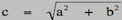.

 Right Triangle Calculator

Right Triangle Calculator
Find the Hypotenuse & Perimeter

Lengths of the two sides:

#### Exercise 7A

Draw a picture of the Web page that would be displayed when this HTML document is loaded. Your drawing should clearly show the layout of the page elements, along with any text, labels, and default values, as they would initially appear in the page.

#### Exercise 7B

What is the purpose of the two lines of text in the HEAD of the page that begin with double slashes (`// Assumes:...` and `// Results:...`)? Would the page behave the same if these two lines of text were removed? Explain your answer.

#### Exercise 7C

Complete the definition of the `Triangulate` function, which accesses the lengths of the two sides entered in the text boxes and uses them to calculate the length of the third side (the hypotenuse). It also calculates the perimeter of the triangle (the sum of all three side lengths) and displays both values in the page. For example, if the user entered `3` and `4` in the text boxes, clicking the button should display the following message: `The hypotenuse is 5, giving a perimeter of 12`.

The next two exercises refer to the following HTML document, which could be used by a person to calculate the "body mass index" of a patient. The Center for Disease Control (CDC) defines body mass index (BMI) using the following formula: BMI = (weight in kilograms) / (height in meters)2.

 Body Mass Index

Body Mass Index
(using the CDC Formula)

#### Exercise 7D

Draw a picture of the Web page that would be displayed when this HTML document is loaded. Your drawing should clearly show the layout of the page elements, along with any text, labels, and default values, as they would initially appear in the page.

#### Exercise 7E

Complete the definition of the `ShowBMI` function, which accesses the weight and height values entered in the text boxes and uses them to calculate the body mass index using the CDC formula. The calculated BMI should be displayed in the `outputDiv` page division in a message of the form: `Your body mass index is XXX`.

### Chapter 9

The next four exercises refer to the following HTML document, which could be used by a person to test whether they have ESP. You may assume that the following images are stored in the same folder as this page: `mystery.gif` (a question mark), `number1.gif`, `number2.gif`, and `number3.gif` (images of the digits 1, 2, and 3, resp.).

 ESP

Do-it-yourself ESP Test

Think of a number between 1 and 3.

Do you have ESP?

#### Exercise 9A

Draw a picture of the Web page that would be displayed when this HTML document is loaded. Your drawing should clearly show the layout of the page elements, along with any text, labels, and default values, as they would initially appear in the page.

#### Exercise 9B

What (if anything) changes in the page when the user clicks on the button? Is the behavior the same each click? Explain your answer.

#### Exercise 9C

Modify the page so that it selects its random number from the range 1-9 (instead of 1-3). You may assume that image files `number4.gif` through `number9.gif` exist in the same folder.

#### Exercise 9D

Augment the above page so that, in addition to its current behavior, a message also appears in `outputDiv` (replacing any text already there). The message should be of the form `The number was X. Did you guess it?` where `X` is the number selected by the page.

### Chapter 11

#### Exercise 11A

Consider the following function that takes two numbers as inputs:
 function Compare(a, b) // Assumes: a and b are numbers // Returns: a message based on the values of a and b { var message; message = '['; if (a < b) { message = message + 'foo'; if (2*a >= b) { message = message + 'bar'; } } else if (a > b) { message = message + 'biz'; } message = message + ']'; return message; }

What value would be returned by each of the following function calls?
• `Compare(8, 5)`
• `Compare(5, 8)`
• `Compare(5, 5)`
• `Compare(5, 20)`

#### Exercise 11B

Design and implement a Web page with three text boxes and a button. When the user clicks the button, the page should display a message identifying whether any of the box contents are identical. The three possible message are 'All values are unique.', 'Two of the three match.', or 'All three are the same.'

#### Exercise 11C

Design and implement a Web page that contains a single button. When the user clicks the button, the page should simulate flipping two coins and display the results, e.g., `You flipped HEADS and TAILS`. If the two flips are identical, then the message should identify this, e.g., `You flipped TAILS and TAILS - they match!`

#### Exercise 11D

Add a counter to your page from Exercise 11C that keeps track of the number of flip matches. Initially, this counter should be 0, and it should be incremented each time matching flips are obtained.

### Chapter 13

#### Exercise 13A

Consider the following function that takes a single number as input:
 function Loopy(number) // Assumes: number is a positive number // Returns: a message based on number { var message; message = ''; while (number > 0) { message = (number % 2) + message; number = Math.floor(number / 2); } return message; }

What value would be returned by each of the following function calls?
• `Loopy(0)`
• `Loopy(1)`
• `Loopy(4)`
• `Loopy(11)`

#### Exercise 13B

Design and implement a Web page with two text boxes (which are to contain positive integers) and a button. When the user clicks the button, the page should display all of the integers in the range 1 to 100 that are divisible by the number in the first box, but not divisible by the number in the second box. For example, if the boxes contained `5` and `10`, then the page should display 5, 15, 25, 35, ..., 95.

#### Exercise 13C

Modify your page from Exercise 13B so that it also displays a count of how many numbers were displayed. For example, `10 numbers are divisible by 5 but not 10.`

### Chapter 15

#### Exercise 15A

Consider the following function that takes a single string as input:
 function Process(word) // Assumes: word is a string // Returns: a string based on word { var i, ch, message; message = 'X'; i = 0; while (i < word.length) { ch = word.charAt(i); message = ch + message + ch; i = i + 1; } return message; }

What value would be returned by each of the following function calls?
• `Process('')`
• `Process('a')`
• `Process('ab')`
• `Process('1234')`

#### Exercise 15B

Consider the page from Exercise 15A. What would happen if the statement `i = i + 1;` were missing from this code? Would the page still load correctly? Would it behave the same when the button was clicked?

#### Exercise 15C

Design and implement a page that contains a text area and button. When the user clicks on the button, any text in the text area is analyzed and statistics displayed in the page. The page should display the number of (non-space) characters in the text area, the number of sentences, and the average number of (non-space) characters per sentence. For simplicity, we will consider any occurrence of a period, question mark, or exclamation mark to denote the end of a sentence.

### Chapter 17

#### Exercise 17A

Consider the following array assignment:
 arr = [ 27, 13.0, 'foo', [1, 2, 3], 'booboo'];

To what value would each of the following expressions evaluate?
• `arr.length`
• `arr`
• `arr[arr.length-1]`
• `arr`
• `arr.length`

#### Exercise 17B

Modify your text statistics page from Exercise 15C so that it also displays the number of words in the text area and the average number of words per sentence. In order to accomplish this, you will need to split the text into individual words using the `split` method. This will also allow for a more accurate count of the number of sentences. A period, exclamation mark, or question mark should only denote a sentence if it appears at the end of a word.

## Solutions to Practice Exercises

#### Exercise 2A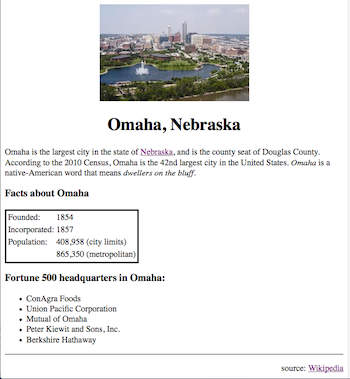#### Exercise 2B

These are comment tags. They are there to document the page, in this case by displaying the file name and a short description. Comments are ignored by the browser, so removing them would not change the appearance of the page in the browser.

#### Exercise 2C

Since the SRC is assigned a relative address (just the file name `omaha.jpg`, without an `http://` prefix), it is assumed to be in the same directory/folder as the Web page. If the image file is not found, then the browser will display a broken image icon. Some broswers may also display the ALT text, `Photo of Omaha Skyline`, with the icon.

#### Exercise 2D

When the user clicks on the link, the browser will take them to the Wikipedia site for Omaha (whose address is `http://en.wikipedia.org/wiki/Omaha,_Nebraska`). That is, the page currently displayed in the browser will be replaced by the Wikipedia page. If the computer was not connected to the Internet, the browser would display an error page, with a message stating that no connection was found.

#### Exercise 4A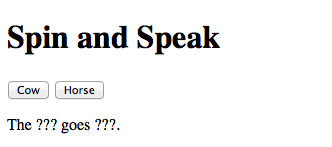#### Exercise 4B

When a button is clicked, each `???` in the page would be replaced by the corresponding animal and sound. For example, if the user clicks on the `Cow` button, then the message would read `The cow goes moo.` Likewise, if they click on the `Horse` button, the message would read `The horse goes neigh.`

#### Exercise 4C

The page would still load the same, displaying the buttons and text as before. When the button is clicked, however, it would cause a JavaScript error, with the resulting error message (in the Error Console) complaining that the specified element is "null" or "not found.". The current contents of the `animalSpan` would remain unchanged.

#### Exercise 4D

The paragraph within the BODY should be changed as follows:


#### Exercise 5A

The scale amount will be an integer ranging from 0 to 4. The smallest value obtainable from `Math.random()` is 0, so `Math.floor(0*5) = Math.floor(0) = 0`. The largest value obtainable from `Math.random()` is 0.9999... (almost, but not quite 1), so `Math.floor(0.999...*5) = Math.floor(4.999...) = 4`.

#### Exercise 5B

Whenever you access the VALUE attribute of a text box, the box contents are treated as a string. Thus, if the user entered `90`, it would be accessed as `'90'`. Then, when you add that string value to a number, e.g., `'90' + 3`, the number is converted into a string an concatenation occurs, e.g., `'90' + 3 → '90' + '3' → '903'`. To avoid this conversion, you should always use `parseFloat` to convert the value in the box into a number. In this case:
`grade = parseFloat(document.getElementById('gradeBox').value);`

#### Exercise 5C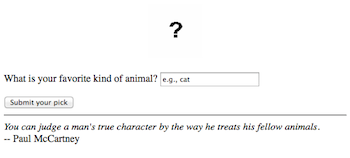#### Exercise 5D

The quote in the page would be replaced by a message that included the animal name. For example, if the user entered `aardvark`, then the message would read `I like aardvarks too!`

#### Exercise 5E

The IMG element within the BODY should be changed as follows:


#### Exercise 5F

Browsers differ in how they would handle this error. Safari generates an error message, stating that the "resource failed to load", and the image is replaced by the broken image icon. Firefox simply ignores the error, leaving the image in the page unchanged.

#### Exercise 7A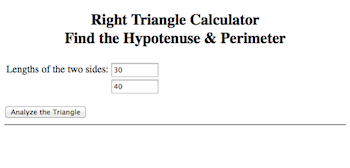#### Exercise 7B

These are JavaScript comments. They are there to document the behavior of the function. Any text preceded by double-slashes is ignored by the JavaScript interpreter, so removing these lines would not change the behavior of the page.

#### Exercise 7C

 function Triangulate() // Assumes: aBox and bBox contain the lengths of the two sides of a right triangle // Results: computes and displays the 3rd side (the hypotenuse) & perimeter in outputDiv { a = parseFloat(document.getElementById('aBox').value); b = parseFloat(document.getElementById('bBox').value); c = Math.sqrt(a*a + b*b); perimeter = a + b + c; document.getElementById('outputDiv').innerHTML = 'The hypotenuse is ' + c + ', giving a perimeter of ' + perimeter + '.'; }

#### Exercise 7D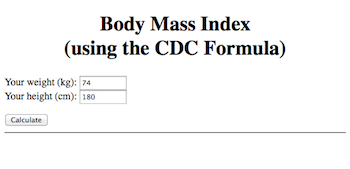#### Exercise 7E

 function ShowBMI() // Assumes: weightBox contains the person's weight in kilograms, // heightBox contains the person's height in centimeters (note: 1 m = 100 cm) // Results: computes body mass index and displays it in outputDiv { kg = parseFloat(document.getElementById('weightBox').value); cm = parseFloat(document.getElementById('heightBox').value); m = cm / 100; bmi = kg/Math.pow(m, 2); document.getElementById('outputDiv').innerHTML = 'Your body mass index is ' + bmi; }

#### Exercise 9A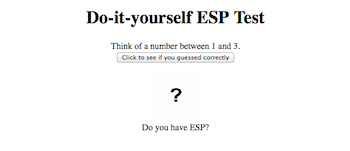#### Exercise 9B

A random integer between 1 and 3 is selected, and the image (`numImg`) is changed to display the corresponding number image (`number1.gif`, `number2.gif`, or `number3.gif`). The behavior is the same each time the button is clicked, but the number selected each time will vary.

#### Exercise 9C

Simply change the `3` in the expression to `9` (and also change the upper limit in the BODY of the page).
`num = Math.floor(Math.random()*9)+1;`

#### Exercise 9D

Add the following assignment at the end of the function:
 document.getElementById('outputDiv').innerHTML = 'The number was ' + num + '. Did you guess it?';

#### Exercise 11A

• `Compare(8, 5) → '[biz]'`
• `Compare(5, 8) → '[foobar]'`
• `Compare(5, 5) → '[]'`
• `Compare(5, 20) → '[foo]'`

#### Exercise 11B

 Classification Page

#### Exercise 11C

 Coin Flip Page

#### Exercise 11D

 // Add at bottom of BODY (before the tag)

Number of matches: 0

// Add at end of IF statement (before the right curly-brace) document.getElementById('matchSpan').innerHTML = parseFloat(document.getElementById('matchSpan').innerHTML)+1;

#### Exercise 13A

• `Loopy(0) → ''`
• `Loopy(1) → '1'`
• `Loopy(4) → '100'`
• `Loopy(11) → '1011'`

#### Exercise 13B

 While Demo Page

Display all integers between 1 and 100 that are divisible by: but not divisible by .

#### Exercise 13C

 While Demo Page

Display all integers between 1 and 100 that are divisible by: but not divisible by .

#### Exercise 15A

• `Process('') → 'X'`
• `Process('a') → 'aXa'`
• `Process('ab') → 'baXab'`
• `Process('1234') → '4321X1234'`

#### Exercise 15B

Without this statement, an infinite loop would occur. The page would still load, but a click of the button would initiate an infinite loop, processing the first number in the sequence (1) over and over. If 1 meets the desired properties, then it will be printed endlessly; if not, then no output would appear but the page would be stuck.

#### Exercise 15C

 Text Stats Page

Enter text here:

#### Exercise 17A

• `arr.length → 5`
• `arr → 13.0`
• `arr[arr.length-1] → 'booboo'`
• `arr → 1`
• `arr.length → 6`

#### Exercise 17B

 Text Stats Page

Enter text here: